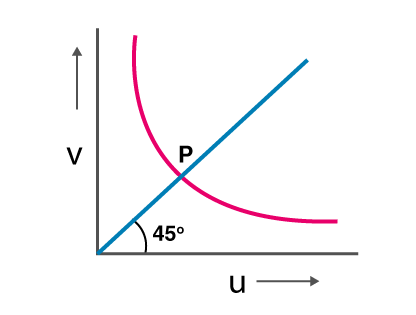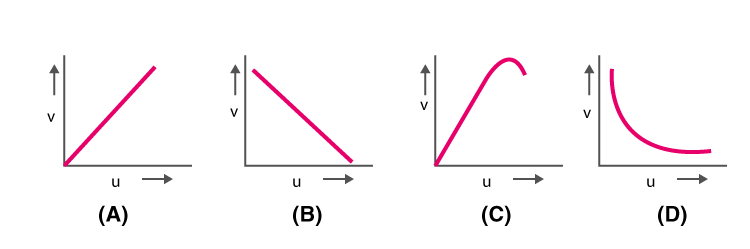## Physics MCQ on Optics for NEET, JEE, Medical and Engineering Exam 2021

MCQ on Optics: Previous trends reveal that NEET/JEE aspirants find Physics as the most challenging section amongst the other sections. But most of them fail to decipher the reason behind this. This particularly can be as the candidates taking up this examination are predominantly medical aspirants with their subject of interest being Biology. Also, sections like Physics are more on theories, laws, numerical as opposed to Biology which is more of fact-based, life sciences and comprising of substantial explanations.In this post we are providing you MCQ on Optics, which will be beneficial for you in upcoming NEET, AIIMS, JEE, WBJEE, Medical and Engineering Exams.

## MCQ on Optics

Q1. What happens to the refractive index on heating the liquid?
a) Increases
b) Decreases
c) Remain unchanged
d) Increases or decreases depending on the rate of heating

(b) Decreases

Q2. Following is the graph explaining the variation of v with respect to u for a mirror. What is the value of points above the marking P on the curve for values of va) Smaller than f
b) Larger than 2f
c) Smaller than 2f
d) Smaller than 2

(b) Larger than 2f

Q3. The image formed by the convex mirror is 1/n times the object and has a focal length f. What is the distance of object from the mirror?
a) (n + 1)f
b) (n – 1)f
c) (n+1/n)f
d) (n-1/n)f

(b) (n – 1)f

Q4. What is hypermetropia?
a) Bad vision due to age
b) Short-sightedness
c) Long-sightedness
d) Formation of a layer on the eye

(c) Long-sightedness

Q5. Calculate the velocity of the light ray in the medium, if the critical angle for TIR from medium to the vacuum is 30°.
a) 2 x 10^8 m/s
b) 1.5 x 10^8 m/s
c) 0.75 x 10^8 m/s
d) 3 x 10^8 m/s

(b) 1.5 x 10^8 m/s

Q6. Which of the following graph is correct when the real image distance v formed by a convex lens is measured for different object distance u.(d)

Q7. Rainbow is an example of which phenomenon?
a) Refraction and scattering
b) Refraction and total internal reflection
c) Dispersion and reflection
d) Dispersion and total internal reflection

(d) Dispersion and total internal reflection

Q8. Which of the following is the combination that is used in the formation of achromatic lenses?
a) 1 convex and 1 plane mirror
b) 2 convex lenses
c) 1 convex and 1 concave lens
d) 2 concave lenses

(c) 1 convex and 1 concave lens

Q9. What is the magnification produced by the plane mirror?
a) Zero
b) +1
c) -1
d) Between 0 and +∞

(b) +1

Q10. How does magnifying power change for an objective lens if the focal length of the objective lens is increased?
a) The microscope will decrease but for the telescope, it will increase
b) The microscope will increase but for the telescope, it decreases
c) The microscope and telescope will increase
d) The microscope and telescope will decrease

(a) The microscope will decrease but for the telescope, it will increase